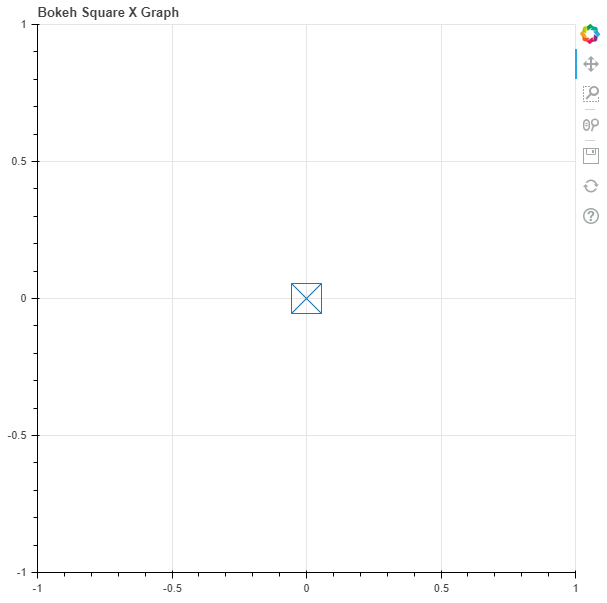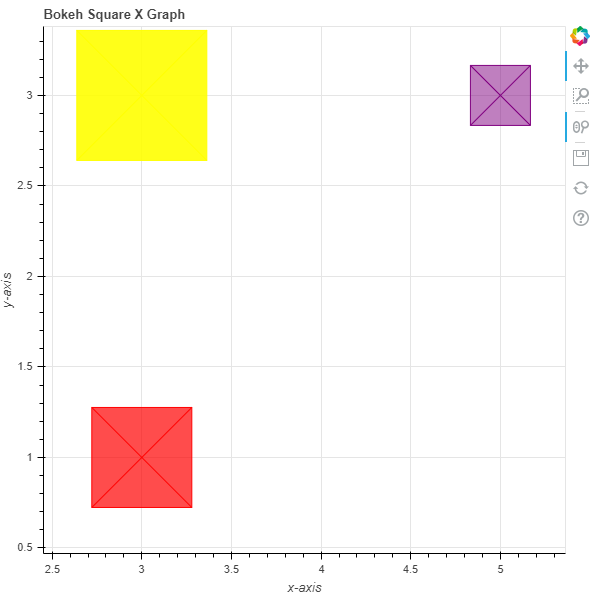Open In App

# Python Bokeh – Plotting Squares with Xs on a Graph

Bokeh is a Python interactive data visualization. It renders its plots using HTML and JavaScript. It targets modern web browsers for presentation providing elegant, concise construction of novel graphics with high-performance interactivity.

Bokeh can be used to plot squares with Xs on a graph. Plotting squares with Xs on a graph can be done using the `square_x()` method of the `plotting` module.

## plotting.figure.square_x()

Syntax : square_x(parameters)

Parameters :

• x : x-coordinates of the center of the square
• y : y-coordinates of the center of the square

Returns : an object of class `GlyphRenderer`

Example 1 : In this example we will be using the default values for plotting the graph.

 `# importing the modules``from` `bokeh.plotting ``import` `figure, output_file, show``       ` `# file to save the model``output_file(``"gfg.html"``)``       ` `# instantiating the figure object``graph ``=` `figure(title ``=` `"Bokeh Square X Graph"``)``     ` `# the points to be plotted``x ``=` `0``y ``=` `0``    ` `# plotting the graph``graph.square_x(x, y, size ``=` `30``, fill_color ``=` `None``)``     ` `# displaying the model``show(graph)`

Output :Example 2 : In this example we will be plotting the multiple squares with various other parameters

 `# importing the modules ``from` `bokeh.plotting ``import` `figure, output_file, show `` ` `# file to save the model ``output_file(``"gfg.html"``) ``      ` `# instantiating the figure object ``graph ``=` `figure(title ``=` `"Bokeh Square X Graph"``) `` ` `# name of the x-axis ``graph.xaxis.axis_label ``=` `"x-axis"``      ` `# name of the y-axis ``graph.yaxis.axis_label ``=` `"y-axis"`` ` `# points to be plotted``x ``=` `[``3``, ``3``, ``5``]``y ``=` `[``3``, ``1``, ``3``]``size ``=` `[``130``, ``100``, ``60``]`` ` `# color value of the square``color ``=` `[``"yellow"``, ``"red"``, ``"purple"``]`` ` `# fill alpha value of the square``fill_alpha ``=` `[``0.9``, ``0.7``, ``0.5``]`` ` `# plotting the graph ``graph.square_x(x, y,``               ``size ``=` `size,``               ``color ``=` `color,``               ``fill_alpha ``=` `fill_alpha) ``      ` `# displaying the model ``show(graph)`

Output :